SEARCH HOMEMath Central Quandaries & QueriesQuestion from Daniel, a student: I tried following an example of yours but I still couldn't figure it out. Here is my question, 2x^2 - 6x + 1 = 0. Any help would be greatly appreciated.Hi Daniel.

Completing the Square method:
To complete the square, what I do first is to get rid of the "a" term (the number at the front) by dividing it through both sides. Then I move the constant term to the other side (changing its sign en route). In your case, I then get this: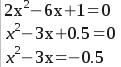Now I complete the square by taking half the "b" term (that's the -3 in front of the x) and squaring that. Half of -3 is -1.5. Square that and I get +2.25. I add it to both sides.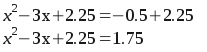At this point, I know the left hand side is a perfect square of the form (x - 1.5)^2. So I can take the square root of both sides, then I can solve for x. Note that when I take the square root, I take both the positive root and negative root, then solve them separately: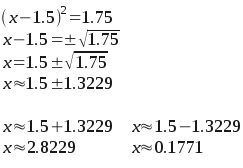So the answers to the equation are x = {0.1771, 2.8229 }.

With such problems, you also always have the option of using the Quadratic Formula. It looks like this: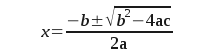In this form, "a" is the number in front of the x^2 term (if there is no number, it is implied to be 1) together with the sign. So in your example, a is 2. Similarly, b and c are the co-efficients of the x term and the constant term, with their signs. So b = -6 and c = 1.

So you can just put those numbers into the Quadratic Formula and simplify.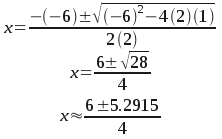That "plus-or-minus" means there are two answers to the question. Here they are side by side. One is the plus version and the other is the minus version.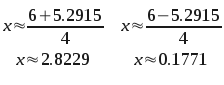Cheers,
Stephen La RocqueMath Central is supported by the University of Regina and The Pacific Institute for the Mathematical Sciences.